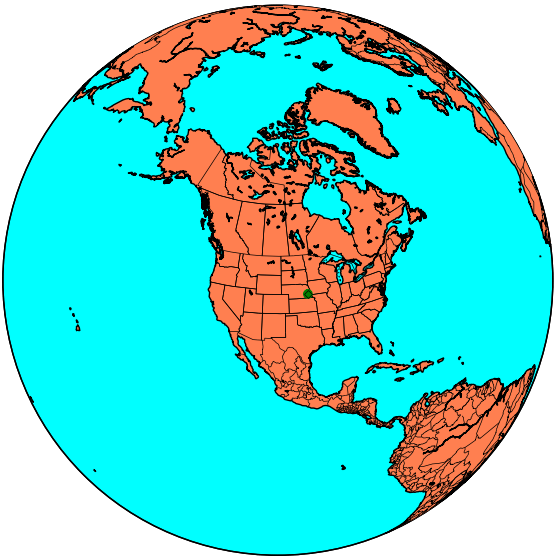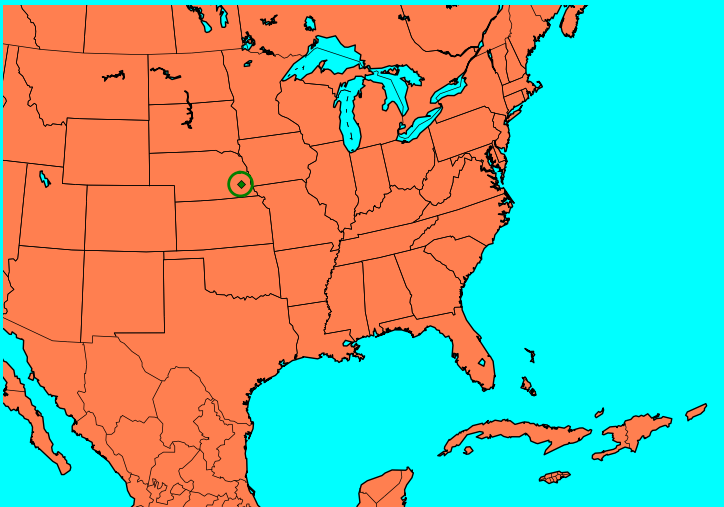# Python-Custom Distance Radius with Basemap

I was working on a project were I needed to identify gaps in coverage based on a location and a service range, which could be different for each service/provider combination. A coverage circle where the radius represents coverage miles would suffice for this project, but I required control over some rendering elements. To complete the project I would have to draw my own coverage circles for each location, thus I needed a function. The function to render each circle is based on the Haversine Formula (https://en.wikipedia.org/wiki/Haversine_formula) if anyone is ever really interested in the steps please let me know and I will include additional details. Otherwise, I will just stick with the code.

### 50 Miles From Memorial Stadium, Lincoln NE, USA

Well I could not think of a really good well known ‘neutral’ location to use in my example, so I picked the most singular location where I live, Memorial Stadium, home of the UNL football team; ‘Smack dab’ in the middle of the U.S.Above is a Basemap plot using an ‘orthographic’ project (ortho for short) with a 50 mile radius drawn around Memorial Stadium. If nothing else, you now know where Memorial Stadium is and Nebraska is (the state containing the green radius).

#### Let’s look at the code

```from mpl_toolkits.basemap import Basemap
import matplotlib.pyplot as plt
import math
from osgeo import ogr, osr
import matplotlib.cm as cmx
import matplotlib.colors as colors```

#### The Haversine Functions

```def createCircleAroundWithRadius(lat, lon, radiusMiles):
ring = ogr.Geometry(ogr.wkbLinearRing)
latArray = []
lonArray = []

for brng in range(0,360):
latArray.append(lat2)
lonArray.append(lon2)

return lonArray,latArray

def getLocation(lat1, lon1, brng, distanceMiles):
lat1 = lat1 * math.pi/ 180.0
lon1 = lon1 * math.pi / 180.0
#R = 6378.1Km
#R = ~ 3959 MilesR = 3959

distanceMiles = distanceMiles/R

brng = (brng / 90)* math.pi / 2

lat2 = math.asin(math.sin(lat1) * math.cos(distanceMiles)
+ math.cos(lat1) * math.sin(distanceMiles) * math.cos(brng))

lon2 = lon1 + math.atan2(math.sin(brng)*math.sin(distanceMiles)
* math.cos(lat1),math.cos(distanceMiles)-math.sin(lat1)*math.sin(lat2))

lon2 = 180.0 * lon2/ math.pi
lat2 = 180.0 * lat2/ math.pi

return lat2, lon2

```

The function createCircleAroundWithRadius expects the lat and long of the center of the circle as well as the miles from the center the radius should be drawn (this could easily be kilometers). This function does little else except iterate over barrings 0-360 degrees. The determination of the radius points is actually done in the function getLocation.

#### Let’s draw something – Orthographic Projection

```memorialStaduimLat = 40.820485
distanceInMiles = 50

#orthographic basemap, center on specified lat and lon
mp = Basemap(projection='ortho', lat_0 = 44, lon_0 = -105, resolution = 'l')

mp.drawstates()
mp.drawcountries()
mp.drawmapboundary(fill_color='aqua')
mp.fillcontinents(color='coral',lake_color='aqua')
mp.drawcoastlines()

#color radius green - seems like a fine color
c = 'g'
# retrieve X and Y radius values using memorial stadium as center point, draw 50 miles out
distanceInMiles)

X,Y = mp(X,Y)
mp.plot(X,Y,marker=None,color=c,linewidth=2)

mp.plot(x,y ,marker='D',color=c,markersize=4)```

#### How about a Mercator Projection

Most of the maps I render do not use the ortho project, but instead use the Mercator (merc) projection. If I would have started with the map below some readers may have been confused as to where in the world we are looking. Orthographic projections can be great when points are spread across a very large area, or when a relevant point is not familiar to an audience (as in this case). Usually, geospatial reports I produce focus on states and counties which is why I often use the Mercator projection. Others may have had different experiences.```westbounds = -105.053514
eastbounds = -95.30829
northbounds = 44.001707
southbounds = 39.999932fig = plt.figure(1)

#merc basemap, center on specified lat and lon, and zoom to bounds
mp = Basemap(projection='merc', lat_0 = 44, lon_0 = -105, ax=fig.gca(),
resolution = 'h', area_thresh = 0.1,
llcrnrlon=westbounds, llcrnrlat=southbounds,
urcrnrlon=eastbounds, urcrnrlat=northbounds)

mp.drawstates()
mp.drawcounties()
distanceInMiles = 50

#color radius green - seems like a fine color
c = 'g'

# retrieve X and Y radius values using memorial stadium as center point, draw 50 miles out
X,Y = mp(X,Y)
mp.plot(X,Y,marker=None,color=c,linewidth=2)

mp.plot(x,y ,marker='D',color=c,markersize=4)

```

I hope this help anyone wanting to indicate coverage areas or distances using circles and Basemap. I am thinking about doing something similar in R if need be. Please let me know if you have any questions or concerns.

Click on a star to rate it!

Average rating 5 / 5. Vote count: 3

No votes so far! Be the first to rate this post.

We are sorry that this post was not useful for you!

Let us improve this post!

Tell us how we can improve this post?

Categories: Geospatial, Python

1.